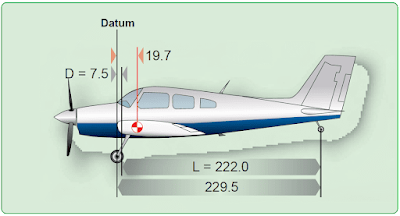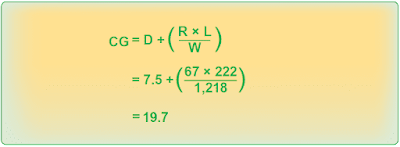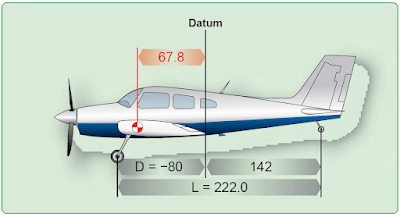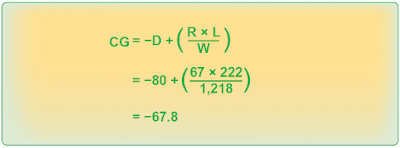# Location of Datum

The location of the datum is not important, but all measurements must be made from the same location.

## Datum Forward of the Main Wheels—Tailwheel Landing Gear

Locating the CG of a tailwheel airplane is done in the same way as locating it for a nosewheel airplane except the formula is

The distance (D) between the datum of the airplane in Figure 1 and the main-gear weighing points is 7.5 inches, the weight of the tailwheel (R) is 67 pounds, and the distance (L) between the main-wheel and the tailwheel weighing points is 222 inches. The total weight of the airplane (W) is 1,218 pounds. Determine the CG by using the formula in Figure 2.Figure 1. The datum of this tailwheel airplane is the wing root leading edgeFigure 2. Determining the CG with datum forward of the main wheels in an airplane with tailwheel landing gear

The CG is 19.7 inches behind the datum.

## Datum Aft of the Main Wheels—Tailwheel Landing Gear

The datum of the airplane in Figure 3 is located at the intersection of the wing root trailing edge and the fuselage. This places the arm of the main gear (D) at –80 inches. The net weight of the tailwheel (R) is 67 pounds, the distance between the main wheels and the tailwheel (L) is 222 inches, and the total net weight (W) of the airplane is 1,218 pounds. Since the datum is aft of the main wheels, use the formula found in Figure 4. The CG is 67.8 inches forward of the datum or 12.2 inches aft of the main-gear weighing points. The CG is in exactly the same location relative to the main wheels, regardless of where the datum is located.Figure 3. Determining the CG with datum forward of the main wheels in an airplane with tailwheel landing gearFigure 4. Determining the CG with datum aft of the main wheels in an airplane with tailwheel landing gear

RELATED POSTS